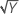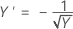# Methods for Fit General Linear Model

Select the method or formula of your choice.

## GLM model

In matrix terms, this is the formula for the general linear regression model:

### Notation

TermDescription
Yvector of responses
Xdesign matrix
βvector of parameters
εvector of independent normal random variables

## Design matrix

General Linear Model uses a regression approach to fit the model that you specify. First Minitab creates a design matrix, from the factors and covariates, and the model that you specify. The columns of this matrix are the predictors for the regression.

The design matrix has n rows, where n = number of observations and several blocks of columns, corresponding to the terms in the model. The first block is for the constant and contains just one column, a column of all ones. The block for a covariate also contains just one column, the covariate column itself. The block of columns for a factor contains r columns, where r = degrees of freedom for the factor, and they are coded as shown in the example below.

Suppose A is a factor with 4 levels. Then it has 3 degrees of freedom and its block contains 3 columns, call them A1, A2, A3.

Level of A A1 A2 A3
1 1 0 0
2 0 1 0
3 0 0 1
4 –1 –1 –1

Suppose factor B has 3 levels nested within each level of A. Then its block contains (3 - 1) x 4 = 8 columns, call them B11, B12, B21, B22, B31, B32, B41, B42, coded as follows:

Level of A Level of B B11 B12 B21 B22 B31 B32 B41 B42
1 1 1 0 0 0 0 0 0 0
1 2 0 1 0 0 0 0 0 0
1 3 –1 –1 0 0 0 0 0 0
2 1 0 0 1 0 0 0 0 0
2 2 0 0 0 1 0 0 0 0
2 3 0 0 –1 –1 0 0 0 0
3 1 0 0 0 0 1 0 0 0
3 2 0 0 0 0 0 1 0 0
3 3 0 0 0 0 –1 –1 0 0
4 1 0 0 0 0 0 0 1 0
4 2 0 0 0 0 0 0 0 1
4 3 0 0 0 0 0 0 –1 –1

To calculate the columns for an interaction term, just multiply all the corresponding columns for the factors and/or covariates in the interaction. For example, suppose factor A has 6 levels, C has 3 levels, D has 4 levels, and Z and W are covariates. Then the term A * C * D * Z * W * W has 5 x 2 x 3 x 1 x 1 x 1 = 30 columns. To obtain them, multiply each column for A by each for C, by each for D, by the covariates Z once and W twice.

## Box-Cox transformation

Box-Cox transformation selects lambda values, as shown below, which minimize the residual sum of squares. The resulting transformation is Y λ when λ ≠ 0 and ln(Y) when λ = 0. When λ < 0, Minitab also multiplies the transformed response by −1 to maintain the order from the untransformed response.

Minitab searches for an optimal value between −2 and 2. Values that fall outside of this interval might not result in a better fit.

Here are some common transformations where Y′ is the transform of the data Y:

Lambda (λ) value Transformation
λ = 2 Y′ = Y 2
λ = 0.5 Y′ =λ = 0 Y′ = ln(Y )
λ = −0.5λ = −1 Y′ = −1 / Y

## Weighted regression

Weighted least squares regression is a method for dealing with observations that have nonconstant variances. If the variances are not constant, observations with:

• large variances should be given relatively small weights
• small variances should be given relatively large weights

The usual choice of weights is the inverse of pure error variance in the response.

The formula for the estimated coefficients is as follows:
This is equivalent to minimizing the weighted SS Error.

### Notation

TermDescription
Xdesign matrix
X'transpose of the design matrix
Wan n x n matrix with the weights on the diagonal
Yvector of response values
nnumber of observations
wiweight for the ith observation
yiresponse value for the ith observationfitted value for the ith observation

## How Minitab removes highly correlated predictors from the regression equation in Fit General Linear Model

To remove highly correlated predictors from a regression equation, Minitab does the following steps:
1. Minitab performs a QR decomposition on the X-matrix.
###### Note

Using the QR decomposition to calculate R2 is quicker than using least-squares regression.

2. Minitab regresses a predictor on all other predictors and calculates the R2 value. If 1 – R2 < 4 * 2.22e-16, then the predictor fails the test and is removed from the model.
3. Minitab repeats steps 1 and 2 for the remaining predictors.

### Example

Suppose a model contains the predictors X1, X2, X3, X4, and X5, and the response Y, Minitab does the following:
1. Minitab regresses X5 on X1-X4. If 1 – R2 is greater than 4 * 2.22e-16 then X5 remains in the equation. X5 passes the test and remains in the equation.
2. Minitab regresses X4 on X1, X2, X3, and X5. Suppose 1 – R2 for this regression is greater than 4 * 2.22e-16 and thus remains in the equation.
3. Minitab regresses X3 on X1, X2, X4, and X5 and calculates the R2 value. X3 fails the test and is removed from the equation.
4. Minitab performs a new QR decomposition on the X-matrix and regresses X2 on the remaining predictors, X1, X4, and X5. X2 passes the test.
5. Minitab regresses X1 on X2, X4, and X5. It fails the test and is removed from the equation.

Minitab regresses Y on X2, X4, X5. The results include a message saying that predictors X1 and X3 cannot be estimated and were removed from the model.

###### Note

You can use the TOLERANCE subcommand with the GLM session command to force Minitab to keep a predictor in the model that is highly correlated with a different predictor. However, lowering the tolerance can be dangerous, possibly producing numerically inaccurate results.

By using this site you agree to the use of cookies for analytics and personalized content.  Read our policy